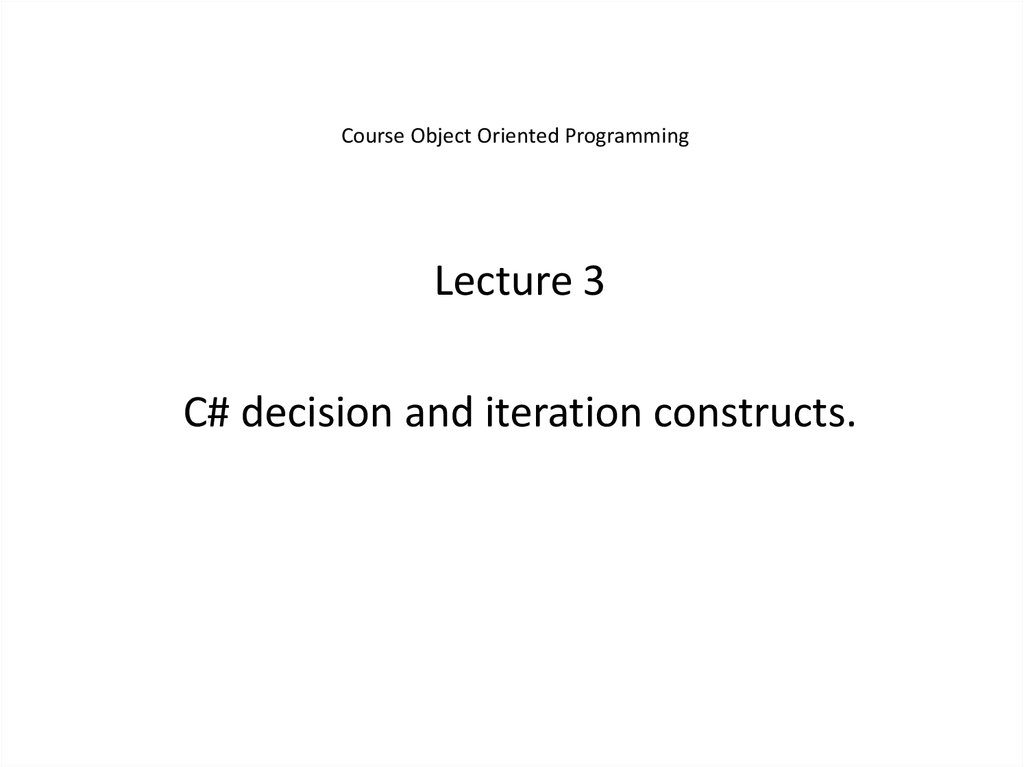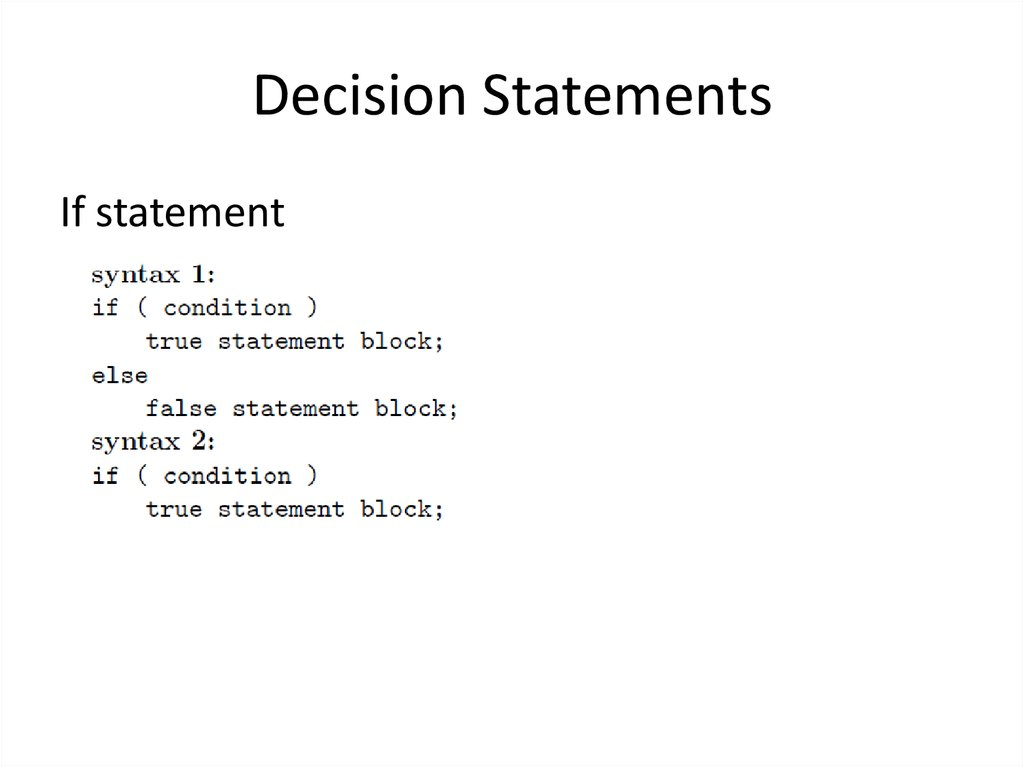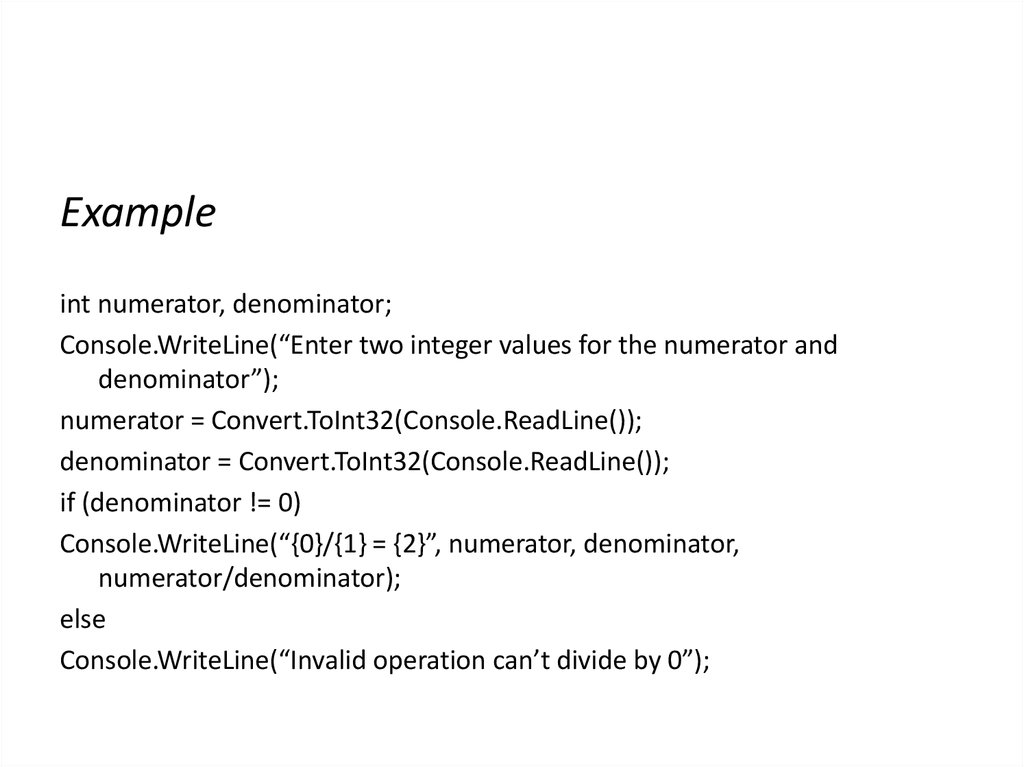# C# decision and iteration constructs

## 1. Course Object Oriented Programming

Lecture 3
C# decision and iteration constructs.

If statement

## 3.

Example
int numerator, denominator;
Console.WriteLine(“Enter two integer values for the numerator and
denominator”);
if (denominator != 0)
Console.WriteLine(“{0}/{1} = {2}”, numerator, denominator,
numerator/denominator);
else
Console.WriteLine(“Invalid operation can’t divide by 0”);

## 4.

The statement body can include more than one statement but make sure
they are group into a code block i.e. surrounded by curly braces.
Example
int x, y, tmp;
if ( x > y)
{
tmp = x;
x = y;
y = tmp;
}

## 5. Nested if Statement

Nested if statements occur when one if statement is
nested within another if statement.
Example
if (x > 0)
if ( x > 10)
Console.WriteLine(“x is greater than both 0 and 10”);
else
Console.WriteLine(“x is greater than 0 but less than or
equal to 10”);
else
Console.WriteLine(“x is less than or equal to 0”);

## 6. if - else - if operator

If a program requires a choice from one of many cases, successive if
statements can be joined together to form a if - else - if ladder.

## 8. Conditional Operator ?:

There is a special shorthand syntax that gives the same result
as
if (expression )
true_statement;
else
false_statement;
syntax: expression ? true_statement : false_statement;
The ?; requires three arguments and is thus ternary. The main
advantage of this operator is that it is succinct.

## 9.

Example
max = x >= y ? x : y;
which is the equivalent of
if ( x >= y)
max = x;
else
max = y;

## 10. Switch Statement

This statement is similar to the if-else-if ladder but is clearer, easier to code
and less error prone.

## 11.

Example
double num1, num2, result;
char op;
Console.WriteLine(“Enter number operator number \n”);
switch(op)
{
case “+”:
result = num1 + num2;
break;
case “-”:
result = num1 - num2;
break;
case “*”:
result = num1 * num2;
break;
case “/”:
if(num2 != 0)
{
result = num1 / num2;
break;
} //else fall through to error statement
default:
Console.WriteLine(“ERROR- invalid operation or divide by 0.0 \n”);
}
Console.WriteLine(“{0} {1},{2} = {3}\n”, num1, op, num2, result);

## 12. Iterative Statements

For statement
While statement
Do while statement
Break statement
Continue statement

## 13. For Statement

A statement or block of statements may be repeated a known number of times using
the for statement. The programmer must know in advance how many times to
iterate or loop through the statements, for this reason the for statement is
referred to as a counted loop.
syntax:
for([initialisation];[condition];[action])
[statement_block];
Square braces indicate optional sections. Initialisation, condition and action can be
any valid C# expression, however, there are common expressions which are recommended for each part.
initialisation: executed once only when the for loop is first entered, usually used to
initialise a counter variable.
condition: when this condition is false the loop terminates.
action: executed immediately after every run through statement_block and typically
increments the counter variable controlling the loop.

## 14.

Example
int x;
for (x = 1; x <= 100; x++)
Console.WriteLine(“{0}”, x);
The above example prints out the numbers from 1 to 100.
Example
int x, sum = 0;
for (x = 1; x <= 100; x++)
{
Console.WriteLine(“{0}”, x);
sum += x;
}
Console.WriteLine(“Sum is {0}”, sum);
Prints the numbers from 1 to 100 and their sum.

for( x = 0, sum = 0; x <= 100; x++)
{
Console.WriteLine(“{0}”, x);
sum += x;
}
for( x = 0, sum = 0; x <= 100; x++)
{
Console.WriteLine(“{0}”, x);
sum += x;
}
for ( ; x < 10; x++)
Console.WriteLine(“{0}”, x);

int i=100,sum=0;
while(i != 0)
sum += i- -;
Console.WriteLine(“sum is {0}”, sum);

## 17. While Statement

In contrast to the for statement, the while statement allows us to loop
through a statement block when we don’t know in advance how
many iterations are required.
syntax:
while( condition )
statement_body;
Example
int sum = 0, i = 100;
while(i != 0) // this condition evaluates to true once i is not equal to 0
sum += i- -; // note postfix decrement operator, why?
Console.WriteLine(“sum is {0}”, sum);
This program calculates the sum of 1 to 100.

## 18.

Like for loops while loops may also be nested.
Example
A program to guess a letter
char ch, letter = “c”, finish = “y”;
while ( finish == “y” || finish == “Y”)
{
Console.WriteLine(“Guess my letter - only 1 of 26!”);
{
Console.WriteLine(“{0} is wrong - try again\n”, ch);
}
Console.WriteLine(“OK you got it \n Lets start again.\n”);
letter += (char)3;
Console.WriteLine(“Do you wish to continue (Y/N)?”);
}

## 19. Do While Statement

In both the for and while statements the test condition is evaluated before the
statement_body is executed. This means that the statement_body might never be
executed. In the do while statement the statement_body is always executed at
least once because the test condition is at the end of the body of the loop.
syntax:
do
{
statement_body;
} while ( condition );
Example
Keep reading in integers until a value between 1 and 10 is entered.
int i;
do
{
} while( i >= 1 && i <= 10);

## 20. Break Statement

When a break statement is encountered in a for, while, do
while or switch statement the statement is immediately
terminated and execution resumes at the next statement
following the loop/switch statement.
Example
for (x = 1; x <= 10 ; x++)
{
if ( x > 4)
break;
Console.Write(“{0} “, x);
}
Console.WriteLine(“Next executed”);
Output is 1 2 3 4 Next executed

## 21. Continue Statement

The continue statement terminates the current iteration of a
for, while or do while statement and resumes execution
back at the beginning of the statement_body of the loop
with the next iteration.
Example
for (x = 1; x <= 5; x++)
{
if (x == 3)
continue;
Console.Write(“{0} “, x);
}
Console.WriteLine(“Finished loop\n”);
output is 1 2 4 5 Finished loop.